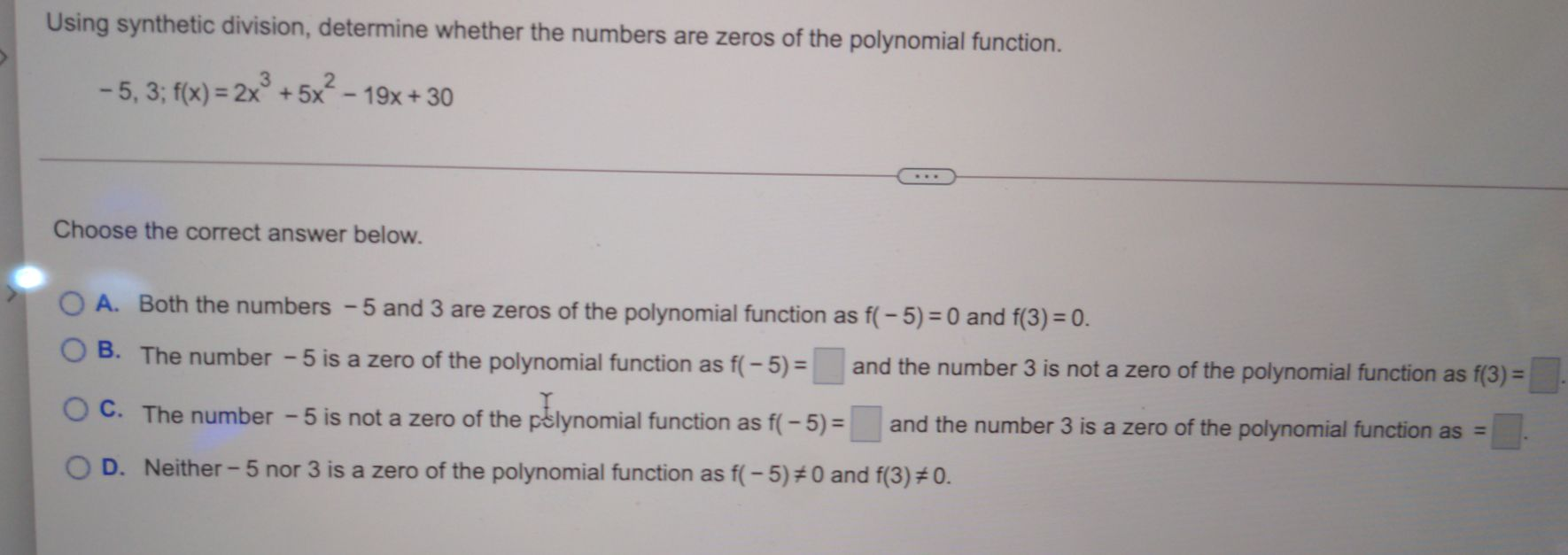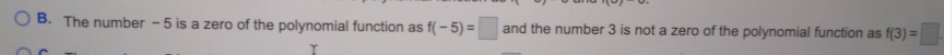### Still have math questions?

Algebra
QuestionUsing synthetic division, determine whether the numbers are zeros of the polynomial function.

$$- 5,3 ; f ( x ) = 2 x ^ { 3 } + 5 x ^ { 2 } - 19 x + 30$$

A. Both the numbers $$- 5$$ and $$3$$ are zeros of the polynomial function as $$f ( - 5 ) = 0$$ and $$f ( 3 ) = 0$$ . B. The number $$- 5$$ is a zero of the polynomial function as $$f ( - 5 ) = \square$$ and the number $$3$$ is not a zero of the polynomial function as $$f ( 3 ) =$$

C. The number $$- 5$$ is not a zero of the polynomial function as $$f ( - 5 ) = \square$$ and the number $$3$$ is a zero of the polynomial function as $$=$$ D. Neither $$- 5$$ nor $$3$$ is a zero of the polynomial function as $$f ( - 5 ) \neq 0$$ and $$f ( 3 ) \neq 0$$ .